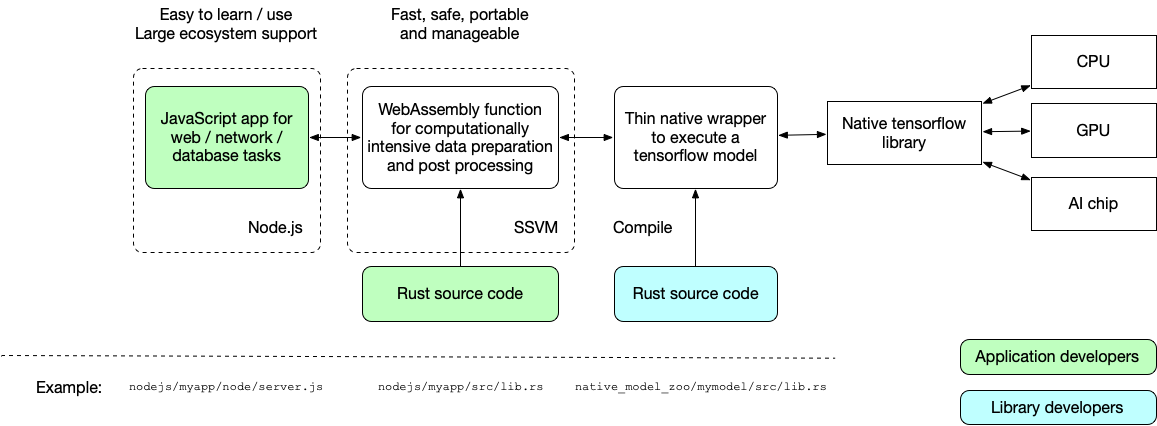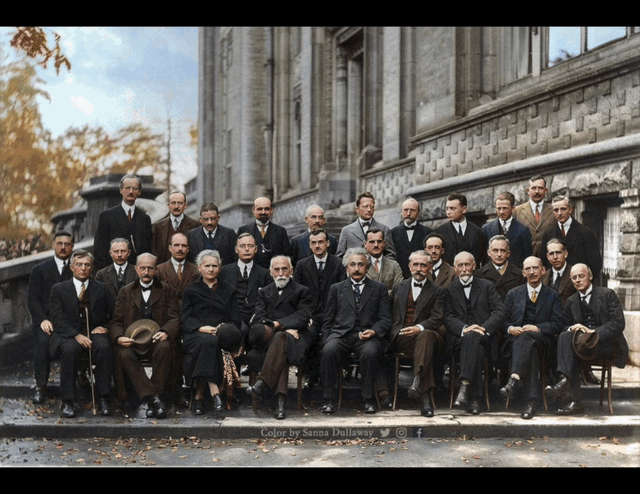# High performance and safe AI as a Service in Node.js

Today’s dominant programming language for AI is Python. Yet, the programming language for the web is JavaScript. To provide AI capabilities as a service on the web, we need to wrap AI algorithms in JavaScript, particularly Node.js.

However, neither Python nor JavaScript by itself is suitable for computationally intensive AI applications. They are high-level, ie, slow, languages with heavy-weight runtimes. Their ease-of-use comes at the cost of low performance. Python got around this by wrapping AI computation in native C/C++ modules. Node.js could do the same, but we have a better way — WebAssembly.

WebAssembly VMs provide tight integration with Node.js and other JavaScript runtimes. They are highly performant, memory safe, secure by default, and portable across operating systems. However, our approach combines the best features of WebAssembly and native code.

## NOTE

This article demonstrates how to call operating system native programs from the SSVM. If you are primarily interested in running Tensorflow models from Rust programs in SSVM, you should check out the SSVM Tensorflow WASI tutorials. It has a much more ergonomic API, and is faster.

## How it works

The Node.js-based AI as a Service application consists of three parts.

• The Node.js application provides web services and calls the WebAssembly function to perform computationally intensive tasks such as AI inference.
• The data preparation, post-processing, and integration with other systems, are done by a WebAssembly function. Initially, we support Rust. The application developer must write this function.
• The actual execution of the AI model is done by native code to maximize performance. This part of the code is very short and is reviewed for security and safety. Application developers just call this native program from the WebAssembly function — much like how native functions are used in Python and Node.js today.Let's look at an example now!

## A face detection example

The face detection web service allows a user to upload a photo, and it displays the image with all pictures marked in green boxes.

The Rust source code for executing the MTCNN face detection model is based on Cetra's excellent tutorial: Face Detection with Tensorflow Rust. We made changes to make the Tensorflow library work in WebAssembly.``````app.post('/infer', function (req, res) {
let image_file = req.files.image_file;
var result_filename = uuidv4() + ".png";

// Call the infer() function from WebAssembly (SSVM)
var res = infer(req.body.detection_threshold, image_file.data);

fs.writeFileSync("public/" + result_filename, res);
res.send('<img src="' +  result_filename + '"/>');
});
``````

As you can see, the JavaScript app simply passes the image data and a parameter called `detection_threshold`, which specifies the smallest face to be detected, to the `infer()` function, and then saves the return value into an image file on the server. The `infer()` function is written in Rust and compiled into WebAssembly so that it can be called from JavaScript.

The `infer()` function flattens the input image data into an array. It sets up a TensorFlow model and uses the flattened image data as input to the model. The TensorFlow model execution returns a set of numbers indicating the coordinates for the four corners of each face box. The `infer()` function then draws a green box around each face, and then it saves the modified image into a PNG file on the web server.

``````#[wasm_bindgen]
pub fn infer(detection_threshold: &str, image_data: &[u8]) -> Vec<u8> {
let mut dt = detection_threshold;
... ...

// Run the tensorflow model using the face_detection_mtcnn native wrapper
let mut cmd = Command::new("face_detection_mtcnn");
// Pass in some arguments
cmd.arg(img.width().to_string())
.arg(img.height().to_string())
.arg(dt);
// The image bytes data is passed in via STDIN
for (_x, _y, rgb) in img.pixels() {
cmd.stdin_u8(rgb as u8)
.stdin_u8(rgb as u8)
.stdin_u8(rgb as u8);
}
let out = cmd.output();

// Draw boxes from the result JSON array
let line = Pixel::from_slice(&[0, 255, 0, 0]);
let stdout_json: Value = from_str(str::from_utf8(&out.stdout).expect("[]")).unwrap();
let stdout_vec = stdout_json.as_array().unwrap();
for i in 0..stdout_vec.len() {
let xy = stdout_vec[i].as_array().unwrap();
let x1: i32 = xy.as_f64().unwrap() as i32;
let y1: i32 = xy.as_f64().unwrap() as i32;
let x2: i32 = xy.as_f64().unwrap() as i32;
let y2: i32 = xy.as_f64().unwrap() as i32;
let rect = Rect::at(x1, y1).of_size((x2 - x1) as u32, (y2 - y1) as u32);
draw_hollow_rect_mut(&mut img, rect, *line);
}
let mut buf = Vec::new();
// Write the result image into STDOUT
img.write_to(&mut buf, image::ImageOutputFormat::Png).expect("Unable to write");
return buf;
}
``````

The `face_detection_mtcnn` command runs the MTCNN TensorFlow model in native code. It takes three arguments, image width, image height, and detection threshold. The actual image data prepared as flattened RGB values is passed in from the WebAssembly `infer()` via `STDIN`. The result from the model is encoded in JSON and returned via the `STDOUT`.

Notice how we passed the model parameter `detection_threshold` the model tensor named `min_size`, and then used the `input` tensor to pass in the input image data. The `box` tensor is used to retrieves the results from the model.

``````fn main() -> Result<(), Box<dyn Error>> {
// Get the arguments passed in from WebAssembly
let args: Vec<String> = env::args().collect();
let img_width: u64 = args.parse::<u64>().unwrap();
let img_height: u64 = args.parse::<u64>().unwrap();
let detection_threshold: f32 = args.parse::<f32>().unwrap();
let mut buffer: Vec<u8> = Vec::new();
let mut flattened: Vec<f32> = Vec::new();

// The image bytes are read from STDIN
for num in buffer {
flattened.push(num.into());
}

// Load up the graph as a byte array and create a tensorflow graph.
let model = include_bytes!("mtcnn.pb");
let mut graph = Graph::new();
graph.import_graph_def(&*model, &ImportGraphDefOptions::new())?;

let mut args = SessionRunArgs::new();
// The `input` tensor expects BGR pixel data from the input image
let input = Tensor::new(&[img_height, img_width, 3]).with_values(&flattened)?;

// The `min_size` tensor takes the detection_threshold argument
let min_size = Tensor::new(&[]).with_values(&[detection_threshold])?;

// Default input params for the model
let thresholds = Tensor::new(&).with_values(&[0.6f32, 0.7f32, 0.7f32])?;
let factor = Tensor::new(&[]).with_values(&[0.709f32])?;

// Request the following outputs after the session runs.
let bbox = args.request_fetch(&graph.operation_by_name_required("box")?, 0);

let session = Session::new(&SessionOptions::new(), &graph)?;
session.run(&mut args)?;

// Get the bounding boxes
let bbox_res: Tensor<f32> = args.fetch(bbox)?;
let mut iter = 0;
let mut json_vec: Vec<[f32; 4]> = Vec::new();
while (iter * 4) < bbox_res.len() {
json_vec.push([
bbox_res[4 * iter + 1], // x1
bbox_res[4 * iter],     // y1
bbox_res[4 * iter + 3], // x2
bbox_res[4 * iter + 2], // y2
]);
iter += 1;
}
let json_obj = json!(json_vec);
// Return result JSON in STDOUT
println!("{}", json_obj.to_string());
Ok(())
}
``````

Our goal is to create native execution wrappers for common AI models so that developers can just use them as libraries.

## Deploy the face detection example

As prerequisites, you will need to install Rust, Node.js, the Second State WebAssembly VM, and the ssvmup tool. Check out the instruction for the steps, or simply use our Docker image. You will also need the TensorFlow library on your machine.

``````\$ wget https://storage.googleapis.com/tensorflow/libtensorflow/libtensorflow-gpu-linux-x86_64-1.15.0.tar.gz
\$ sudo tar -C /usr/ -xzf libtensorflow-gpu-linux-x86_64-1.15.0.tar.gz
``````

To deploy the face detection example, we start from the native TensorFlow model driver. You can compile it from Rust source code in this project.

``````# in the native_model_zoo/face_detection_mtcnn directory
\$ cargo install --path .
``````

Next, go to the web application project. Run the ssvmup command to build the WebAssembly function from Rust. Recall that this WebAssembly function performs data preparation logic for the web application.

``````# in the nodejs/face_detection_service directory
\$ ssvmup build
``````

With the WebAssembly function built, you can now start the Node.js application.

``````\$ npm i express express-fileupload uuid

\$ cd node
\$ node server.js
``````

The web service is now available at port 8080 of your computer. Try it with your own selfies or family and group photos!

## The TensorFlow model zoo

The native Rust crate `face_detection_mtcnn` is a fragile wrapper around the TensorFlow library. It loads the trained TensorFlow model, known as a frozen saved model, sets up inputs for the model, executes the model, and retrieves output values from the model.

In fact, our wrapper only retrieves the box coordinates around detected faces. The model actually provides a confidence level for each detected face and positions of eyes, mouth, and nose on each face. By changing the retrieve tensor names in the model, the wrapper could get this information and return to the WASM function.

If you would like to use a different model, it should be fairly easy to follow the example and create a wrapper for your own model. You just need to know the input and output of tensor names and their data types.

To achieve this goal, we create a project, called the native model zoo, to develop ready-to-use Rust wrappers for as many TensorFlow models as possible.

## What's next

In this article, we demonstrated how to implement a real-world AI as a Service use case in Node.js using Rust and WebAssembly. Our approach provides a framework for the entire community to contribute to a “model zoo”, which can serve as the AI library for more application developers.

In the next tutorial, we will review another TensorFlow model for image classification and demonstrate how the wrapper can be extended to support a whole class of similar models.

RustJavaScripttensorflowWebAssemblyNode.jsAI as a serviceFaaSAIFace detection